# Workshop2 - Data processing with functions

In this workshop we are going to learn how to use functions to solve a practical problem. We are going to build a data processing pipeline which simple functions as building blocks.

We are going to build the solution piece by piece to learn and better understand how different Scala constructs help us to create configurable and elastic logic.

We will learn :

• How to compose more powerful functions from simple functions
• What is loan pattern and how to separate reading a file from file processing
• How to use map-reduce as a powerful configurable skeleton for data processing.

## Reading Data - Part 1

We are going to work with the following data

``````Login,Product,Price,Date
user1,tv,3000,01-02-2016
user2,tv,3000,02-02-2016
user1,console,1500,02-02-2016
user3,book,30,03-02-2016
user3,poster,15,03-02-2016
user1,stereo,1000,03-02-2016
user3,tv,3000,04-02-2016
user1,mouse,25,10-02-2016
``````

As the first thing in our workshop we want to read from the file only lines where login is user1.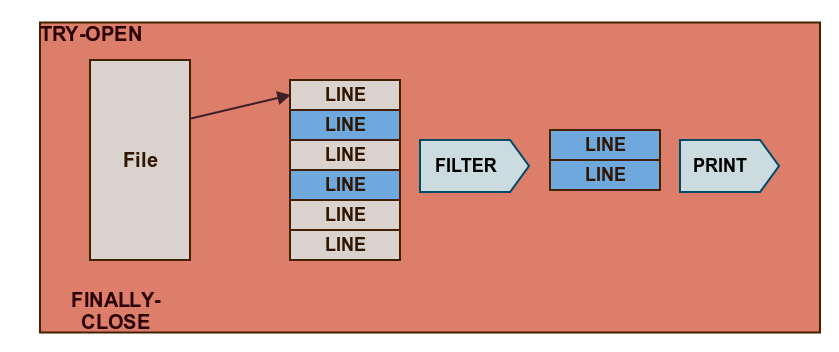In demo part you can see an example on how to filter values in scala. This knowledge will be useful later.

``````def demonstration(l:List[Int]):List[Int]= l.filter(e=>e>7)
``````

### Exercises

Exercise : filter and keep even numbers

``````  def filter_1(l:List[Int]):List[Int]= ???
//expected
filter_1(List(1,2,3,4,5,6,7,8,9,10))==List(2,4,6,8,10)
``````

Exercise : left only numbers which are in someSet

`````` val someSet=Set(3,7,10,50)
def filter_2(l:List[Int]):List[Int]= ???
//expected
filter_2(List(1,2,3,4,5,6,7,8,9,10))==List(3,7,10)
``````

Exercise : read file and keep lines where Login=user1

There is a prepared method which uses scala.io.Source

`````` def readFile(): Unit = {
val source: BufferedSource = Source.fromURL(getClass().getResource("/fpjava/purchases.csv"))
try {
source.getLines()
// ???  //extract lines with user="user1"
.foreach(println)
} finally {
source.close()
}
}
``````

Expected result :

``````user1,tv,3000,01-02-2016
user1,console,1500,02-02-2016
user1,stereo,1000,03-02-2016
user1,mouse,25,10-02-2016
``````

Additional Exercise : divide list according to predicate

• First element of the tuple - Even Numbers
• Second element of the tuple - Odd Numbers
``````def additional(l:List[Int]): (List[Int], List[Int])
//expected
additional(List(1,2,3,4)) == (List(2,4),List(1,3))
``````

## Configuring filtering with Currying - Part 2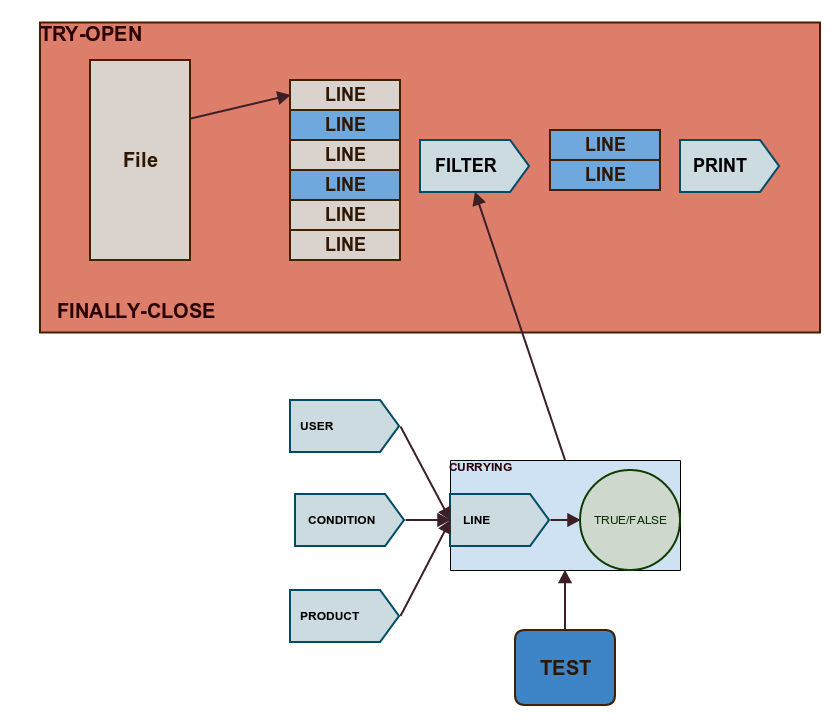To gain better testability we can actually define predicate function outside of the file loop and then just use it there. This way we can test it independently from the main program.

In Scala we can define methods with multiple parenthesis which allow us to partially apply arguments or in other words to specify part of arguments later.

``````def demonstration2(l:List[Int])(predicate:Int=>Boolean):List[Int]= l.filter(predicate)
def demonstration3(predicate:Int=>Boolean)(l:List[Int]):List[Int]= l.filter(predicate)

println(demonstration2(List(1,2,3,4,5))(e=>e>3)==List(4,5))

val preparedFilter = demonstration3(e=>e>5)_
println(preparedFilter(List(3,4,5,6,7,8))==List(6,7,8))
``````

This way we can preconfigure filtering function for a given list or for given predicate

### Exercises

Exercise : create predicate for User1

As a warm up create standard predicate which checks if in line there is a condition fulfilled Login=user1

``````val user1Predicate:String => Boolean = ???
``````

Additional Exercise : create predicates factory

Then create predicates factory which can create predicate for defined field

``````val predicateForField : (Int,String) => String => Boolean = ???

val generatedCsvPredicate = predicateForField(0,"user1")
val generatedCsvPredicateForTV = predicateForField(1,???)
val generatedCsvPredicateForDate = predicateForField(???,"02-02-2016")
``````

Exercise : use predicate in the file loop

`````` source.getLines()
//Exercise
.filter(???)
.foreach(println)
``````

## Testing - Part 3

Now when predicate functionality is created independently from the file loop we can test it very easy and then inject preconfigured logic into loop as a strategy

`````` def readFile(filePath:String)(p:String=>Boolean): Unit = {
val source: BufferedSource = Source.fromURL(getClass().getResource(filePath))
try {
source.getLines()
.filter(p)
.foreach(println)
} finally {
source.close()
}
}
``````

Exercise : write tests for predicate functionality

You can write unit test :

`````` "Produced Predicate" should "check proper field with proper value" in {
val line= ???

val predicate: (String) => Boolean = ???

predicate(line) shouldBe(true)
}
``````

Or Property test

`````` property("'predicateForField' should generate predicate for given field and value"){
forAll(listOfValues){vs=>
val value=vs.head
val csvLine=vs.mkString(",")
println(csvLine)
}
}
``````

or both!

## Custom Structures - Part 4

We want to decouple activity of handling file (opening/closing/catching exceptions) from an activity of processing its content.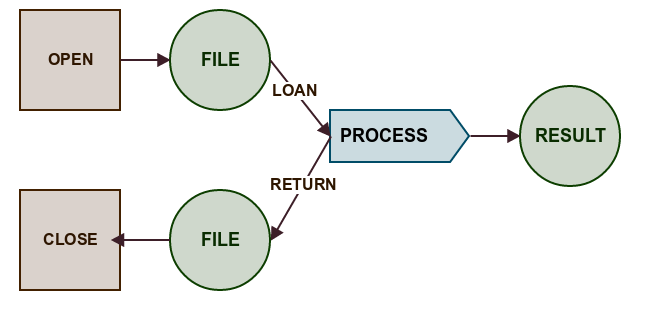Scala provides a very expressive syntax for such constructions but first we need to complete some exercises to better understand those constructions nature.

First take a look at the example :

`````` def demonstration1(value:String)(action : String => Unit)={
val calculationResult=s"result of some calcualtions based on '\$value'"
action(calculationResult)
}
``````

When we have method defined with multiple parenthesis when the last one is function we can call it in standard way

``````  demonstration1("someValue")(result=>println(result))
``````

We can also create execution block

``````demonstration1("someValue")({result=>println(result)})
``````

And when we have execution block defined we can omit parentheses which allow us to write scala instructions in a way that they looks like native language constructs.

``````demonstration1("someValue"){result=>println(result)}
demonstration1("someValue"){result=>
println(result)
}
``````

### Exercises

Exercise : write custom fold

``````def customFold(seq:Seq[Int])(op:(Int,Int)=>Int):Int= ???
//so we can call
val customFoldResult=customFold(List(1,2,3,4,5)){(a:Int,b:Int) =>
a+b
}
``````

Exercise : write hero lookup function

In this example observe that argument given in the first pair of parentheses can be transformed before it is passed into the function defined later. We will use it in file loan pattern

``````case class SuperHero(name:String, power:String)
val heroes:Map[String,SuperHero] = Map("Spiderman"->SuperHero("Spiderman","Web"),"J23" -> SuperHero("J23","Being Undercower"))

def heroFight(heroName:String)(action:SuperHero => Unit)= ???

//so we can call
heroFight("J23"){hero:SuperHero =>
println(s"hero \${hero.name} is fighting with \${hero.power}")
} // should display : hero J23 is fighting with Being Undercower
``````

Additional Exercise : write custom while

definition :

`````` def customWhile(condition: => Boolean)(action : =>Unit):Unit= ???
``````

usage:

``````var i=0
customWhile(i<5){
println(s" i = \$i")
i=i+1
}
``````

Additional Exercise : write custom if

Observe the difference between statement and expression

``````def customIfExpression[A](condition : => Boolean)(action: =>A)(elseAction: =>A): A = ???

def customIfStatement(condition : => Boolean)(action: =>Unit)(elseAction: =>Unit): Unit = ???
``````

## Loan a File - Part 5

Now it's time to prepare infrastructure to loan file content to a process function. For educational purposes we will use List[String] as a file content.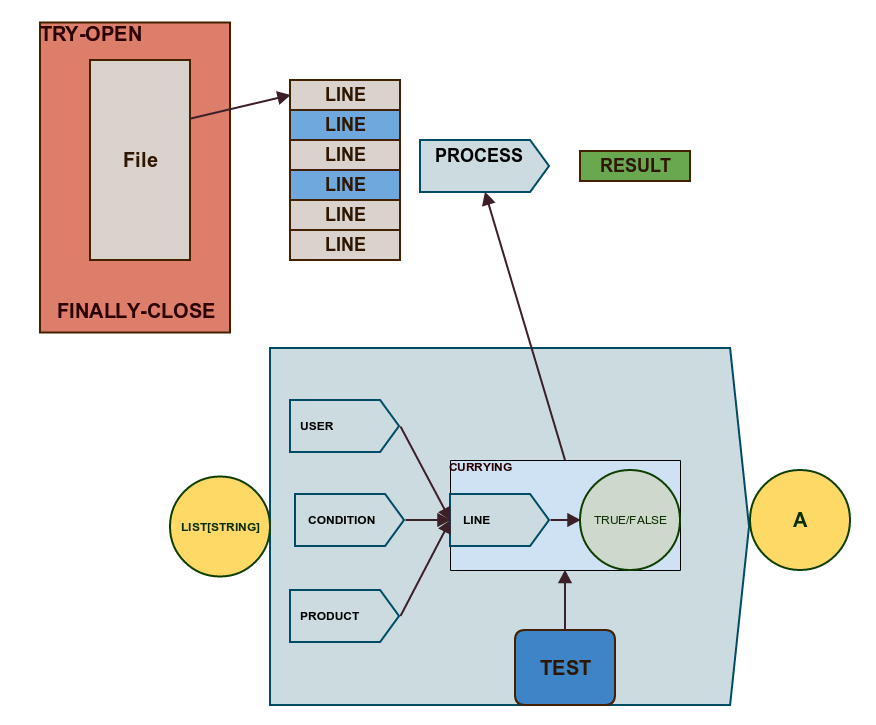Exercise : write loan method

Declaration looks like this :

``````def readFile[B](filePath:String)(process:List[String]=>B): Unit = ???
``````

And the usage

`````` readFile("/fpjava/purchases.csv"){lines=>
lines.filter(generatedCsvPredicate).foreach(println)
}
``````

Additional Exercise : write loan curried function

In this exercise we will also use type alias to improve readability.

``````type Predicate=String=>Boolean
``````

implement process function with filtering configured through currying

``````lazy val processContent:Predicate=>List[String]=>List[String]= ???
``````

implement loan curried function

`````` val readFileCurried: (String) => ((List[String]) => List[String]) => List[String] = ???
``````

## Map File Content - Part 6

For now we only used filtering in processing part. We are going to transform each line into particular field which will be very handy for further processing.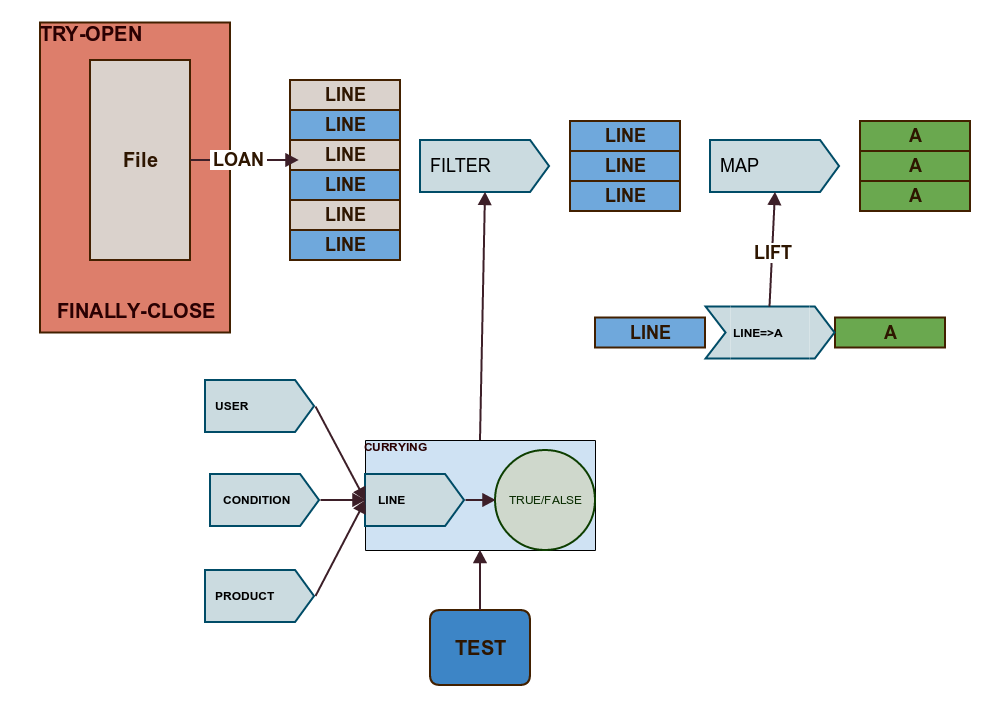First of all in our pipeline we are operating on a list of values . Let's create some aliases to simplify the code.

An alias for a function operating on a list level will be called Transformation

``````type FilePath=String
type Text=String
type Transformation=List[Text]=>List[Text]
val processContent:Predicate=>Transformation= p => lines => lines.filter(p)
val readFileCurried: (FilePath) => (Transformation) => List[String] = ...
``````

It would be very usefull to use simple function which operates on a simple values and somehow move them into Lists level

``````val extractPrice:Text => Text
``````

Exercise : lift simple function to list level

implement extract price function and also special lift function which transform simple function to function which operate on lists level.

``````lazy val extractPrice:Text => Text = ???
lazy val lift: (Text=>Text) => (List[Text]=>List[Text]) = ???
``````

Additional Exercise : lift other structures

Write lift function for other structures - you should spot some similarity.

`````` def liftGeneric[A,B](f:A=>B):List[A]=>List[B] = ???

def liftOption[A,B](f:A=>B):Option[A]=>Option[B] = ???

def liftTry[A,B](f:A=>B):Try[A]=>Try[B] = ???
``````

ADDITIONAL HARDCORE EXERCISE : lift with Functors

If you finish additional exercise most likely you noticed that implementation for all structure is somehow similar - it looks like some common abstraction is hiding there.

Implement general lift function which uses Functor defined for a given structure.

``````  import cats.std.all._
implicit val listFunctor:Functor[List]=Functor[List]
implicit val optionFunctor:Functor[Option]=Functor[Option]

def liftFunctor[A,B,F[_]](f:A=>B)(implicit functor:Functor[F]): F[A] => F[B] = ???
``````

usage :

``````val lifted: (List[Text]) => List[Text] = liftFunctor[Text,Text,List](extractPrice)
println(lifted(List("user1,tv,100","user2,console,200"))==List("100","200"))

val liftedOption: (Option[Text]) => Option[Text] =liftFunctor[Text,Text,Option](extractPrice)
println(liftedOption(Some("user1,tv,100"))==Some("100"))
``````

## Reduce File Content - Part 7

Now we want to have possibility to compute final result which can be A single value, A List, A Map, Anything. To achieve this we need reduce functionalityIn this exercise we are going to implement reduce function with usage of pattern matching and recursion. So to prepare yourself for exercise take look at the following

#### Pattern Matching

Following function returns second element

``````  def secondElement[A](l:List[A])= l match {
case first::second::tail => second
case _ => throw new RuntimeException("list has less than two elements!")
}
``````

#### Internal functions

Internal functions allow you to implement internal recursion with different method signature that in external function.

`````` def external(prefix:String, suffix:String) :String = {
def internal(middle:String) = prefix+middle+suffix

internal("internal method exercise")
}
``````

Exercise : implement reduction of integers

``````def reduce(combine:(Int,Int)=>Int)(l:List[Int]) :Int = ???
//usage
val addAll: (List[Int]) => Int = reduce((element1, element2)=>element1+element2)
``````

Additional Exercise : implement generic fold function

``````def fold[A,B](zero:B)(l:List[A])(f:(B,A)=>B):B= ???
//usage
fold(0)(List(1,2,3,4,5))(_+_) == 15
``````

## Unified Map Reduce - Part 8

What we achieved :

We have a generic functional library

``````  object FunctionalLibrary{
def liftGeneric[A,B](f:A=>B):List[A]=>List[B]
def liftOption[A,B](f:A=>B):Option[A]=>Option[B]
def liftTry[A,B](f:A=>B):Try[A]=>Try[B]
def fold[A,B](zero:B)(f:(B,A)=>B)(l:List[A]):B
def reduce[A](combine:(A,A)=>A)(l:List[A])
}
``````

We have set of independent predicates

``````val predicateForField : (Int,String) => String => Boolean =
(index,expectValue)=>line=>line.split(",")(index)==expectValue

val whenUser1 = predicateForField(0,"user1")
val generatedCsvPredicateForTv = predicateForField(1,"tv")
val generatedCsvPredicateForDate = predicateForField(3,"02-02-2016")
``````

And infrastructure to customize processing pipieline

`````` //MAP-REDUCE
lazy val extractPrice:String => String = line => line.split(",")(2)
val priceToInt =  extractPrice andThen (_.toInt)
val sumOfPricesFromUser1  =  filterLines(whenUser1) andThen MapReduce(priceToInt)(reduce[Int](_+_))

def processFile[C](path:String)(mapReduce:List[String]=>C):C={
``````

It's time to implement composable skeleton factory for map-reduce function

Exercise : implement map-reduce combiner

``````object MapReduce{
def apply[A,B,C](fmap:A=>B)(reduce:List[B]=>C) : List[A] => C = ???
}
``````

Additional Exercise : count products

To see how elastic this approach is define flow which counts how many which products was purchased

``````lazy val extractProduct:String => String = ???

val countOccurences: (List[String]) => Map[String, Int] = ???

val occurencesOfProduct = MapReduce(extractProduct)(countOccurences)
``````

# Homework

• Write tests for as many functions defined during those workshops as possible.
• Which phases of our flow can be easily executed in parallel?